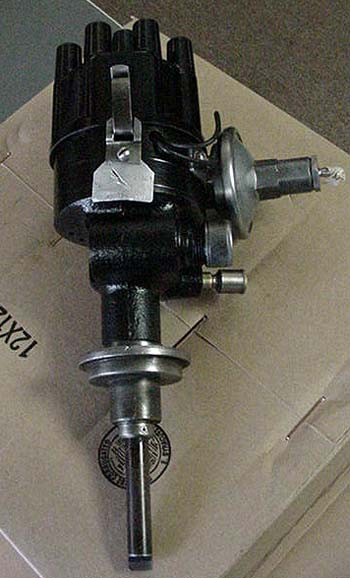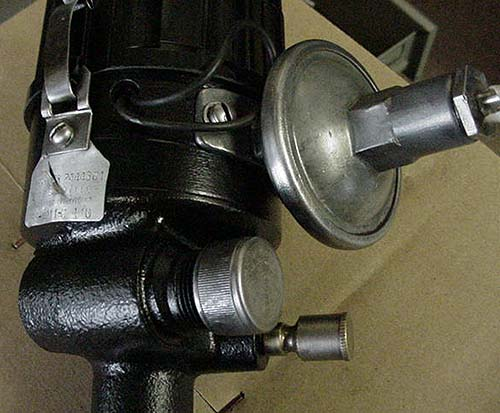Revised 02/15/13

Summary of Letter Car Distributors

Identification
 Distributor Number 1955 C300 1AZ4001G, 1658989 300B 1704309, IBK-4301C 300C 1689325, IBK-4304 300D IBS 4007A; 1841515 300D Fuel Injection IBS 4008 300E IBS-4010, 1842805 300F Autolite IBS-4011; Chrysler number 1889568 300G Autolite IBS-4011; Chrysler number 1889568 300H 2098313, IBS-4011AS 300J 2098620 (Auto-lite) 300K 2444361, IBS-4011C 300K ram 2444364, IBS-4011D 300L 2444683 (Chrysler), IBS-4006K (Prestolite)

Specifications
 1955 C-300 Engine Idle Setting: 600 RPM, Ignition Timing: 100 B.T.D.C. Note: Reduce engine idle to 500 RPM, set ignition at 100 B.T.D.C. and reset engine idle back to 600 RPM. Ref: 1955 Chrysler Service Manual Page 285. Additional note: Distributor components are identical to those found on many other 1955 Chrysler product V-8 engines EXCEPT for the governor weight spring set, cam and stop plate and vacuum chamber. Ref 1955-58 Chrysler Passenger Car Parts List. Automatic Advance Curve (distributor degrees and distributor RPM) 00 at 300 to 400 RPM 00 to 3.50 at 400 RPM 30 to 50 at 500 RPM 70 to 90 at 800 RPM Vacuum Advance Curve (distributor degrees at inches of mercury) 10 at 5.5” to 6.5” 50 to 6.50 at 10” 60 to 80 at 11”

 300B Automatic Advance Curve (distributor degrees and RPM) 00 at 350 to 450 00 to 4.250 at 450 2.80 to 4.80 at 500 70 to 90 at 1200 Vacuum Advance Curve (distributor degrees at Inches of Mercury) Start at 7” to 8” 20 at 8.5” to 9.5” 60 at 11.25” to 12.75” 70 to 90 at 13.5”

 300C Advance Curves IBK 4304; 1689325 Automatic Advance Curve (distributor degrees and RPM) 00 at 250 to 450 00 to 20 at 450 40 to 60 at 875 90 to 110 at 2250 Vacuum Advance Curve (distributor degrees at Inches of Mercury) Start at 7.5” to 8.75” 20 at 8.75” to 10.2” 60 at 11.75” to 13.4” 100 to 120 at 18”

 300D Advance Curves IBK 4304; 1689325 Automatic Advance Curve (distributor degrees and RPM) 00 at 220 to 490 00 to 20 at 490 20 to 40 at 750 3.50 to 4.50 at 850 80 to 110 at 2400 Vacuum Advance Curve (distributor degrees at Inches of Mercury) 00 at 7.5” to 8.5” 4.50 to 6.50 at 11” 100 to 120 at 16”

 300E Automatic Advance Curve (distributor degrees and RPM) 00 at 310 to 490 00 to 20 at 490 3.50 to 5.50 at 800 60 to 80 at 1500 8.50 to 10.50 at 2300 Vacuum Advance Curve (distributor degrees at Inches of Mercury) 00 at 7.5” to 9.1” 60 to 90 at 13” 11.50 to 14.50 at 18.2”

 300F, 300G Automatic Advance Curve (distributor degrees at distributor RPM) 00 at 325 to 475 00 to 4.30 at 475 4.50 to 6.50 at 640 90 to 110 at 2400 Vacuum Advance Curve (distributor degrees at Inches of Mercury) 00 at 7.2” to 8.9” 4.50 to 7.50 at 12” 7.50 to 10.50 at 14.5”

 300H Automatic Advance Curve (distributor degrees at distributor RPM) 00 at 325 to 475 00 to 30 at 475 4.50 to 6.50 at 640 90 to 110 at 2400 Vacuum Advance Curve (distributor degrees at Inches of Mercury) 00 at 7.2” to 8.9” 4.50 to 7.50 at 12” 7.50 to 10.50 at 14.5”

 300J Automatic Advance Curve (distributor degrees at distributor RPM) 00 at 525 to 675 00 to 30 at 675 4.50 to 6.50 at 910 Vacuum Advance Curve (distributor degrees at Inches of Mercury) 00 at 6” to 9” 4.50 to 7.50 at 12” 7.50 to 10.50 at 14.3”

 300K Automatic Advance Curve (distributor degrees at distributor RPM) 00 at 325 to 475 00 to 40 at 475 4.50 to 6.50 at 640 90 to 110 at 2400 Vacuum Advance Curve (distributor degrees at Inches of Mercury) 00 at 7.2” to 8.9” 4.50 to 7.50 at 12” 7.50 to 10.50 at 14.5”
 300K Ram Automatic Advance Curve (distributor degrees at distributor RPM) 00 at 525 to 675 00 to 30 at 675 4.50 to 6.50 at 910 Vacuum Advance Curve (distributor degrees at Inches of Mercury) 00 at 6” to 9” 4.50 to 7.50 at 12” 7.50 to 10.50 at 14.3”

 300L Automatic Advance Curve (distributor degrees at distributor RPM) 00 at 325 to 475 00 to 40 at 475 4.50 to 6.50 at 640 90 to 110 at 2400 Vacuum Advance Curve (distributor degrees at Inches of Mercury) 00 at 7.2” to 8.9” 4.50 to 7.50 at 12” 7.50 to 10.50 at 14.5”

Thanks to Rich Barber for some of the data.

Photos of a K distributorA beat up tag, but still legible.The tach drive mechanism is contained in the casting below the tag, with a metal cap screwed on.
Manual transmission Ks used the drive.
Automatic transmission Ks didn't use the drive and had a cap for dirt prevention.
The brass cap is the grease cup for the distributor shaft bearing.

Sharp eyes might notice the L distributor curve matches the F.
Perhaps this is not surprising since both use the same cam shaft.

Back to Tech List